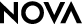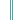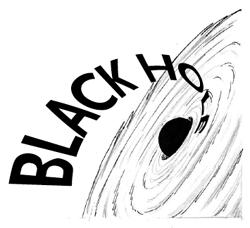Monster of the Milky WayStudent Handout

Getting Really DenseIn the first part of this activity, you crushed an aluminum ball more and more and measured its increasing density. Now you will do some calculations to determine how much more dense that ball would become if you could continue to compress it. Then you will determine the size of your aluminum ball black hole's event horizon—the point past which nothing returns.

Going down to ... one centimeter
Imagine that you could overcome electric forces and compress your aluminum into a sphere one centimeter in diameter. What would its density be? Using the graph you created, calculate its value using D = M/V.

And farther down to ... one millimeter
What is the density of your ball of aluminum if it is squeezed into a sphere 1 millimeter in diameter? Calculate its value using D = M/V.

And farther down to ... a neutron
What would the density of your model be if you could compress your aluminum into a sphere the size of a neutron (the average radius of a neutron is equal to10-15 m)? Calculate its value using D = M/V.

And finally down to a ... black hole
The mass of a black hole is squeezed into a sphere with a radius very close to zero that results in a volume that is very close to 0 cm3. What is the density of a black hole using this volume? Calculate its value using D = M/V.

Any mass can become a black hole if there is enough force to make it small enough. Imagine that your aluminum foil star model became a black hole. Determine the radius of its event horizon, called the Schwarzschild radius. The expression that determines the radius of the event horizon is:

R = 2GM/c2

where R is the radius of the event horizon, M is the mass of the black hole in kg, G is the universal gravitational constant, and c is the speed of light. (G = 6.67 x 10-11m3/kg-sec2 and c = 3 x 108 m/sec)

The event horizon if our sun were to become a black hole
Our sun is not massive enough to ever go supernova and produce a black hole. It will evolve into a red giant star and later will become a white dwarf star, slowly cooling over billions of years to a black ball of carbon atoms. But if our sun could become a black hole:

• What would the size of its event horizon be (Msun = 2 x 1030 kg) ? Use the equation above to calculate its value.

• What would life be like on Earth if the sun was replaced by a black hole with the mass of the sun?

• Would Earth be sucked into the black hole?

-->